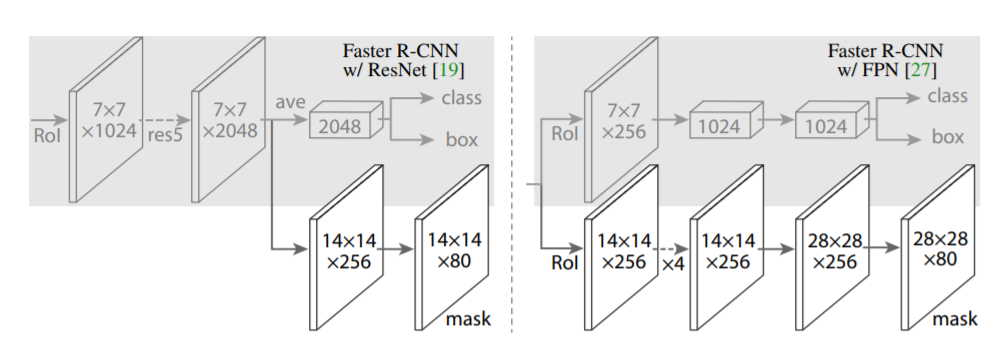# 关于改进HRNet的论文阅读笔记

## Deep High-Resolution Representation Learning for Human Pose Estimation

### 模型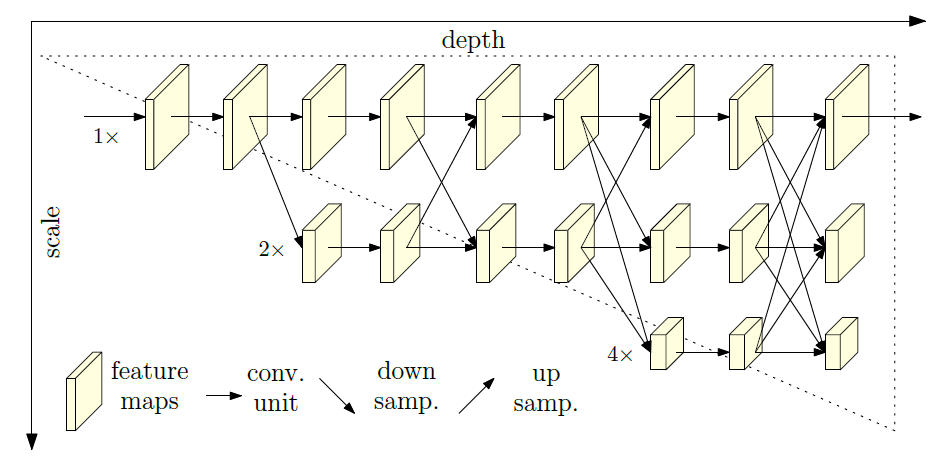\begin{equation}
\begin{array}
\mathcal{N}_{11} & \rightarrow ~~ \mathcal{N}_{22} &\rightarrow ~~ \mathcal{N}_{33} & \rightarrow ~~ \mathcal{N}_{44}
\end{array}
\end{equation}

\begin{equation}
\begin{array}
\mathcal{N}_{11} & \rightarrow ~~ \mathcal{N}_{21} &\rightarrow ~~ \mathcal{N}_{31} & \rightarrow ~~ \mathcal{N}_{41} \\
& \searrow ~~ \mathcal{N}_{22} &\rightarrow ~~ \mathcal{N}_{32} & \rightarrow ~~ \mathcal{N}_{42} \\
& &\searrow ~~ \mathcal{N}_{33} & \rightarrow ~~ \mathcal{N}_{43} \\
& & & \searrow ~~ \mathcal{N}_{44}
\end{array}
\end{equation}

\begin{equation}
\begin{array}
\mathcal{C}^1_{31} & \searrow &\nearrow ~~ \mathcal{C}^2_{31} & \searrow &\nearrow ~~ \mathcal{C}^3_{31} & \searrow \\
\mathcal{C}^1_{32} & \rightarrow ~~ \mathcal{E}^1_{3} & \rightarrow ~~ \mathcal{C}^2_{32} & \rightarrow ~~ \mathcal{E}^2_{3} & \rightarrow ~~ \mathcal{C}^3_{32} & \rightarrow ~~ \mathcal{E}^3_{3}\\
\mathcal{C}^1_{33} & \nearrow &\searrow ~~ \mathcal{C}^2_{33} & \nearrow &\searrow ~~ \mathcal{C}^3_{33} & \nearrow\\
\end{array}
\end{equation}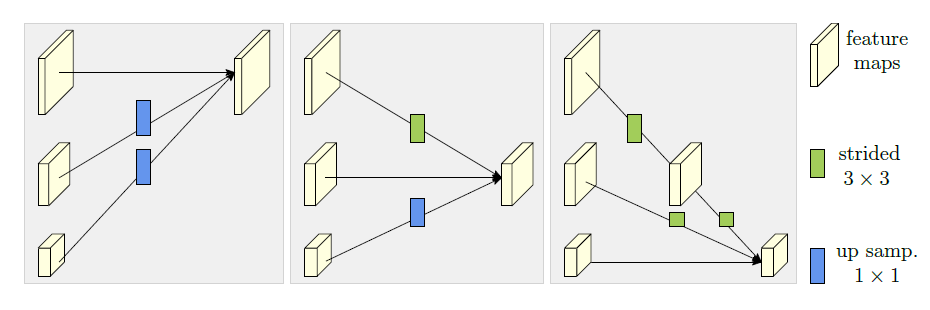### 介绍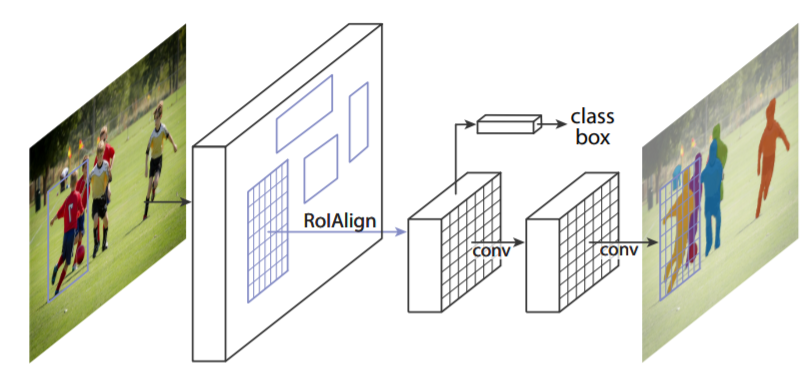### 结构

##### RoIAlign

RoIPool分割feature map时采用的是四舍五入取整，会带来误差。RoiAlign不进行取整，用双线性插值计算每个采样点处于的PoI区域四个格点上取值的和或均值。如下图所示。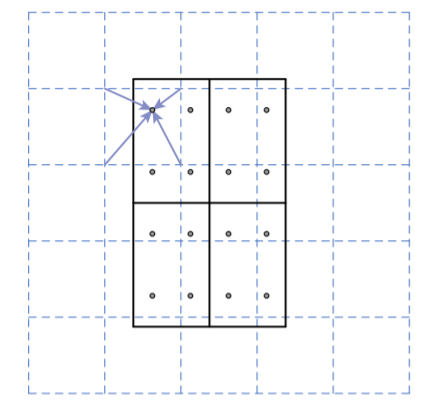##### 网络结构